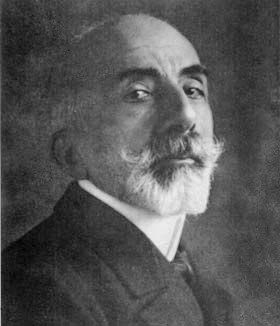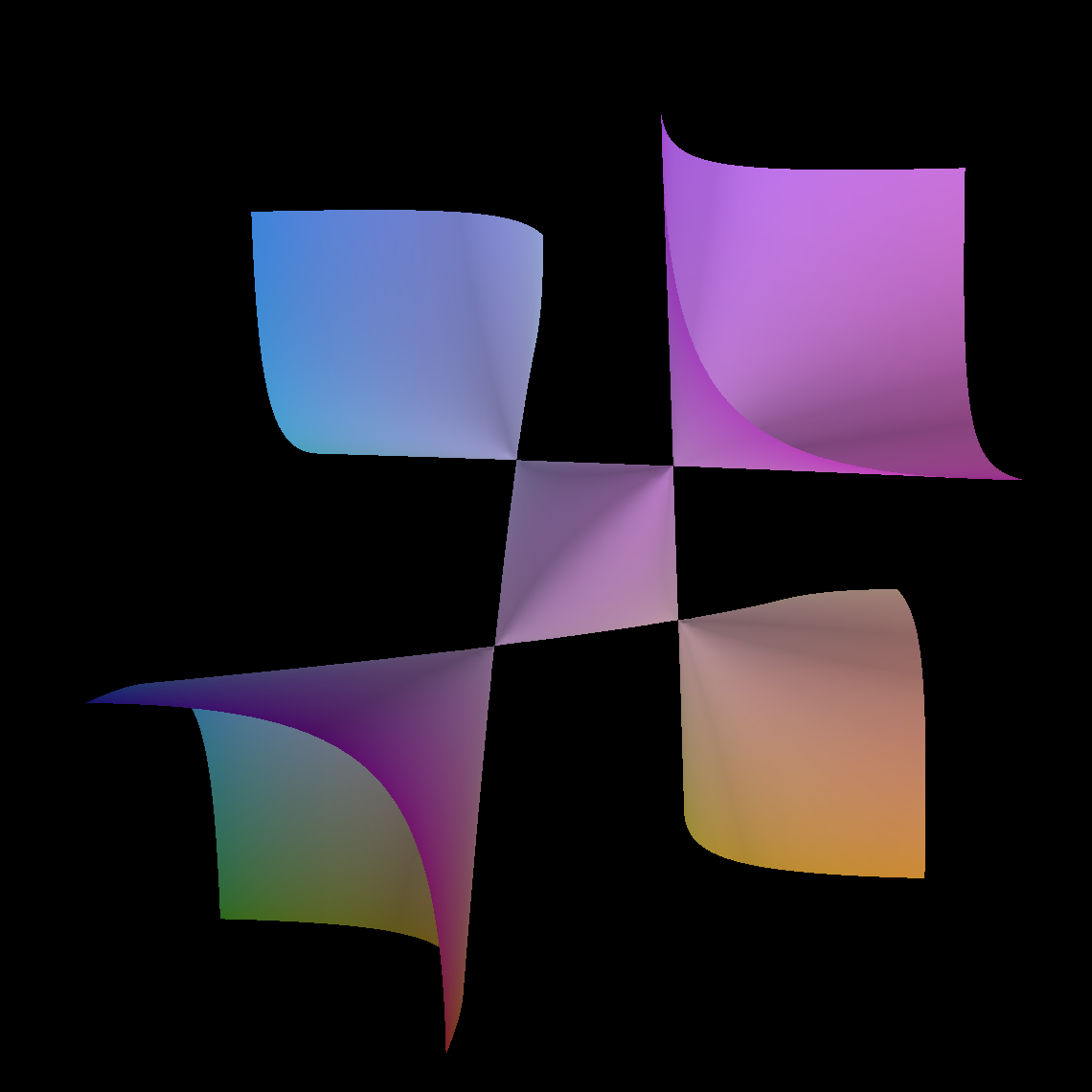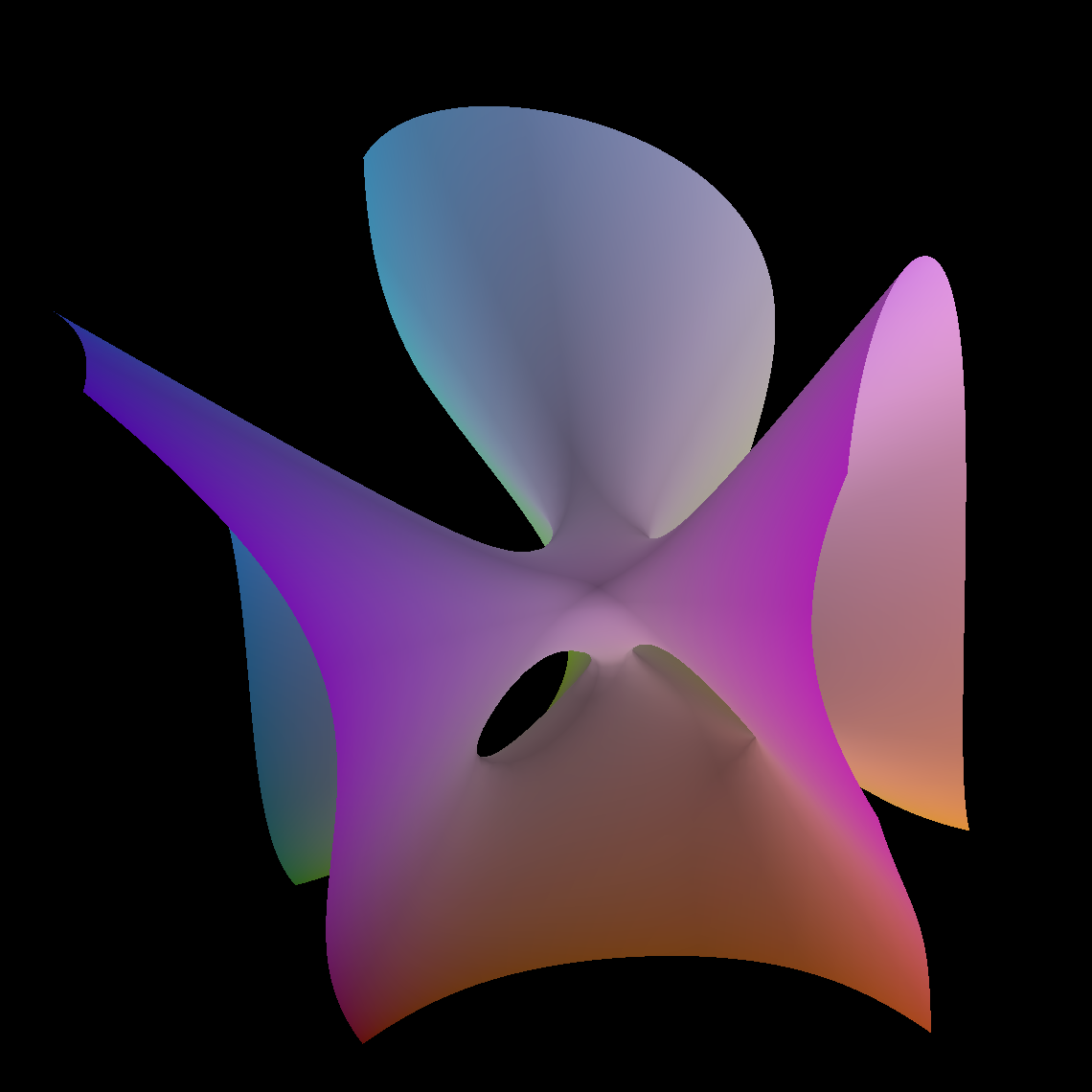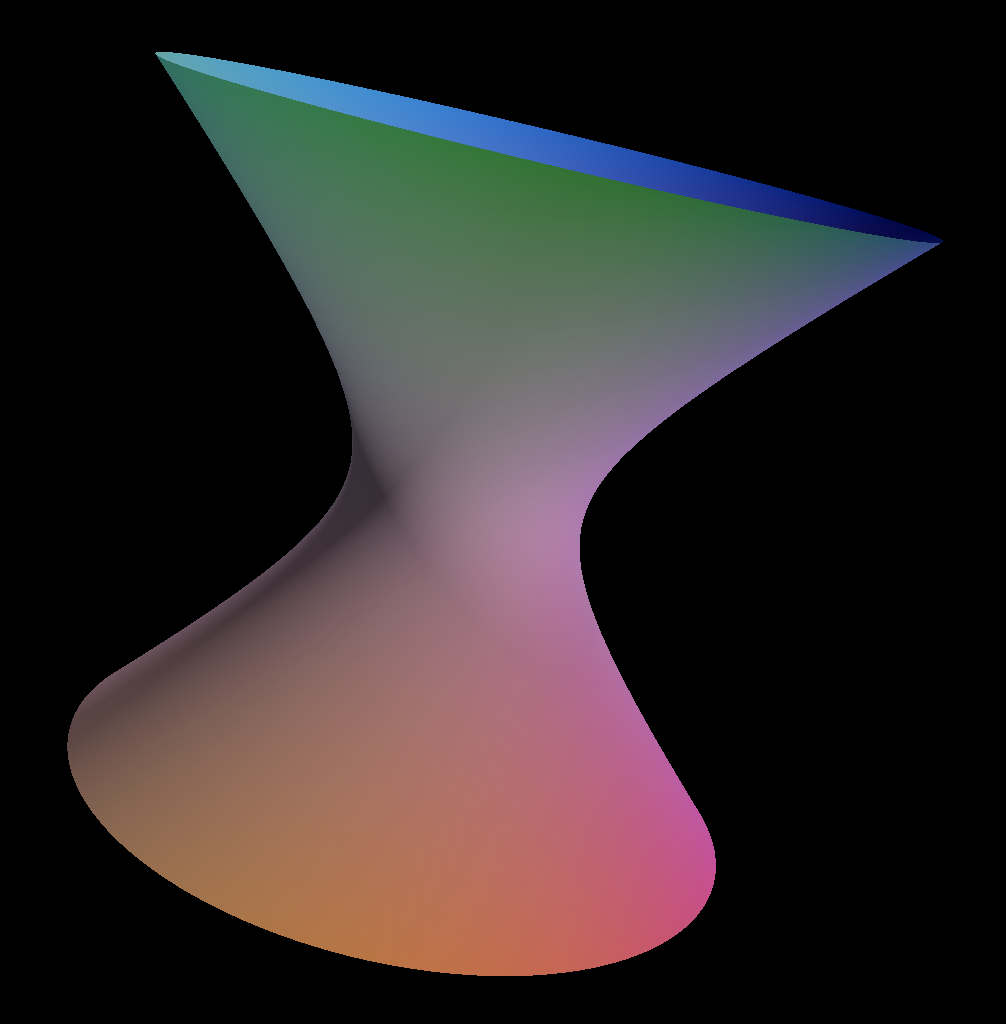This really should have been a well-established series by now, but so far there was only one prior installment. Should I write some of these retroactively maybe?

Thorsten Beckmann and I have a new paper on the arXiv, titled Homological projective duality for the Segre cubic, intended as a semi-expository contribution for a proceedings volume. It was great fun writing it, and let me try to informally and with pictures convey some of the things that made writing it great fun, in the hope of convincing you to take a closer look at the paper.

## The Segre cubicBack in 1887, Corrado Segre, one of the frontrunners of the Italian school, studied a cubic 3-fold, written most conveniently as the vanishing locus \begin{equation} \left\{ \begin{aligned} \sum_{i=0}^5x_i&=0 \\ \sum_{i=0}^5x_i^3&=0 \\ \end{aligned} \right. \end{equation} in $\mathbb{P}^5$ (so that it's really a cubic hypersurface in the hyperplane given by the first equation). It is the cubic 3-fold with the highest number of isolated singularities: there are 10 nodal singularities. One can beautifully describe aspects of its geometry in terms of its (large) automorphism group: $\mathrm{S}_6$.

By taking further hyperplane sections it also gives rise to convenient descriptions of some classical cubic surfaces:

Cayley cubic• obtained by a hyperplane section of the form $x_i-x_j=0$
• this is the cubic surface with the highest number of isolated singularities: there are 4 nodal singularities
• its automorphism group is also interesting: it is $\mathrm{S}_4$, which is the largest amongst the parameter-free singular cubic surfaces with finite automorphism group

Clebsch cubic• obtained by a hyperplane section of the form $x_i=0$
• this is the cubic surface with the highest number of real lines: all 27 lines on the cubic can be defined over the real numbers, making this surface a standard example to illustrate the geometry of cubic surfaces
• its automorphism group is also interesting: it is $\mathrm{S}_5$, which is the second-largest (the largest automorphism group arises for the Fermat cubic, and it's a semidirect product of $\mathrm{S}_4$ with $(\mathbb{Z}/3\mathbb{Z})^3$

## Homological projective geometryIn classical projective geometry the notion of duality plays an important role. One standard example for the projective plane is are the dual statements that through every 2 distinct points goes a unique line and that every 2 distinct lines intersect in a unique point. For (embedded) projective varieties there exists a whole theory of projective duality, showing how one can recover a variety from its dual, defined as the closure of the points in the dual projective space corresponding to tangent hyperplanes at smooth points.

I like to study varieties through their derived categories, and the theory of projective duality was generalised by Kuznetsov to a notion of homological projective duality in 2007. Nowadays classical constructions in projective geometry, such as cones and joins, have an incarnation on the level of derived categories, making them applicable to settings far beyond the usual projective varieties, and this theory goes by the name of homological projective geometryMy favourite basic example in projective duality is a (smooth) quadric hypersurface, which is projective dual to another smooth quadric hypersurface. On the level of derived categories an interchange between hypersurfaces and double covers arises (essentially because there are 1 or 2 spinor bundles depending on the parity of the dimension).

Surely, going from a degree 2 equation to a degree 3 equation shouldn't make life too hard?! Turns out it does! If one needs convincing of the rich and complicated nature of cubic hypersurfaces, one can check out the book-in-progress by Daniel Huybrechts.

Now, there does exist a framework that can handle homological projective duals for smooth cubic hypersurfaces. But its output is highly non-trivial. Rather than work with smooth cubics, one can also consider singular cubics, and look for an interesting resolution of singularities, and have fun with the geometry of the original cubic vs. that of the singularity, and that of its (homological) projective dual.

This reduces the complexity of the machinery, and the "easiest" case is the most singular case, that of the Segre cubic.

## What did we do?

The Segre cubic and one of its resolutions plays an essential role in Cheltsov, Kuznetsov, Shramov: Coble fourfold, $\mathfrak{S}_6$-invariant quartic threefolds, and Wiman-Edge sextics. The resolution is a $\mathbb{P}^1$-bundle over the del Pezzo surface $\operatorname{Bl}_4\mathbb{P}^2$ of degree 5. And one of the first results in homological projective duality is a description of homological projective duality for projective bundles, so we can just apply that machinery!

We end up with an explicit construction of the homological projective dual (as a resolution of the Coble fourfold), which

• ties together lots of super-classical (19th century!) algebraic geometry in terms of 21st century methods
• gives a nice showcase of methods in homological projective duality: I for one think having this example worked out when I was learning the theory would have been useful
• features cameos of many explicit and interesting varieties: there is a role for cubic surfaces (and the Cayley and Clebsch surface from above in particular), del Pezzo double planes, the blowup of $\mathbb{P}^2$ in 4 points, the Segre cubic threefold, the Castelnuovo–Richmond–Igusa quartic threefold, the Coble fourfold, elliptic curves, and their derived categories.
• allows for an easy and explicit discussion of derived fiber products

Let me know if you have any questions after reading this, or reading the paper. We've tried to make it accessible (its goal is to be somewhat expository after all), but if things are not clear we gladly improve them.